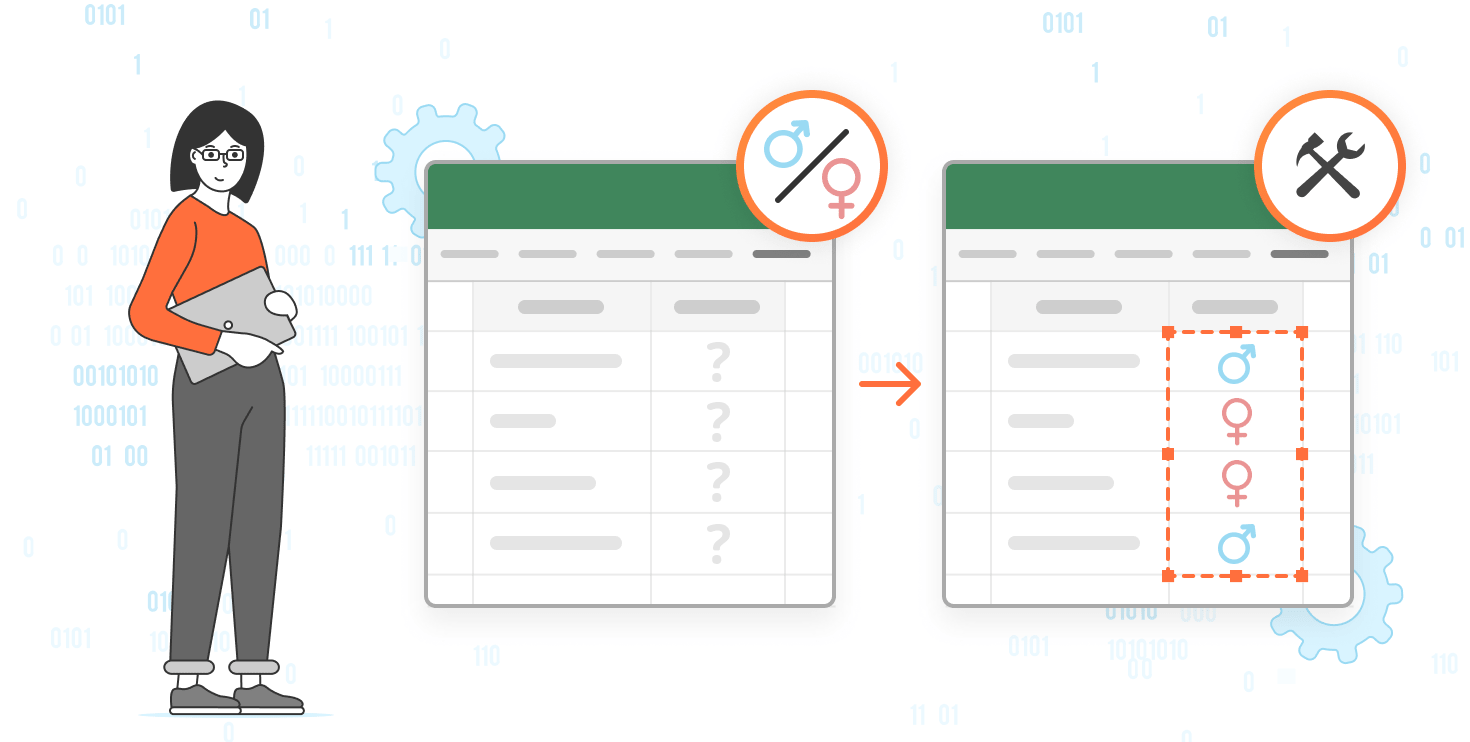# Generate personalized gender-based greetings with ONLYOFFICE macro

18 July 2023

Addressing people correctly is crucial in communication as it shows respect, inclusivity, and professionalism. In this article, we show you how to build a macro in ONLYOFFICE Docs that utilizes the Genderize.io API to generate proper personalized greetings.Genderize.io is an API service that predicts the gender associated with given names. It provides developers with an easy way to integrate gender identification functionality into their applications or scripts. By sending a name as a parameter to the Genderize API, users receive a response containing the predicted gender and additional information such as the confidence level of the prediction. Genderize.io simplifies the process of determining the gender associated with a given name.

## Building the macro

We start by initializing the oWorksheet variable, which holds a reference to the active sheet. Having access to the active sheet enables us to manipulate the data within the spreadsheet:

``````const oWorksheet = Api.GetActiveSheet()
``````

Next, we initialize the ROW variable to 2, representing the starting row of the data in column A. This variable keeps track of the current row being processed:

`````` let ROW = 2
``````

To process each row, we have the checkRow function. Its main objective is to iterate through each row and handle the processing logic. Inside the checkRow function, we retrieve the name value of the current row by using the oWorksheet.GetRange(A\${ROW}).GetText() statement. This retrieves the text value of the cell in column A for the current row:

``````function checkRow() {
const name = oWorksheet.GetRange(`A\${ROW}`).GetText()
}
``````

If the retrieved name is empty, it indicates the end of the data. In this case, we call the reload function, which is responsible for reloading the sheet. On the other hand, if the retrieved name is not empty, we proceed to call the makeRequest function, passing the name as a parameter:

``````   function checkRow() {
const name = oWorksheet.GetRange(`A\${ROW}`).GetText()
if (name === '') {
}
makeRequest(name)
}
``````

The purpose of the makeRequest function is to send an AJAX request to the Genderize API:

``````function makeRequest(name) {
\$.ajax({
url: `https://api.genderize.io?name=\${name}`,
dataType: 'json',
}).done(successFunction)
}
``````

Once the response is received from the Genderize API, the successFunction is invoked. Within the successFunction, we first check if the probability of the gender determination is greater than 0.9. If it is, we assign the appropriate title based on the gender value from the API response:

``````function successFunction(data) {
if (data.probability > 0.9) {
let title = data.gender === 'male' ? 'Mr' : 'Ms'
}
}
``````

To generate the personalized greeting, we use the oWorksheet.GetRange(B\${ROW}).SetValue(…) method. This allows us to set the value in column B of the current row. After successfully processing the current row, we increment the ROW variable and then call the checkRow function again. This recursive approach ensures that we continue to iterate through each subsequent row until we reach the end of the data:

``````function successFunction(data) {
if (data.probability > 0.9) {
let title = data.gender === 'male' ? 'Mr' : 'Ms'
oWorksheet.GetRange(`B\${ROW}`).SetValue(`Dear \${title} \${data.name}!`)
}
ROW++
checkRow()
}
``````

Once the end of the data is detected, we invoke the reload function. The purpose of this function is to reload the sheet, triggering a recalculation of all formulas. To achieve this, we use setInterval to periodically execute the Api.asc_calculate(Asc.c_oAscCalculateType.All) function, which recalculates all the formulas in the sheet:

``````    function reload() {
Api.asc_calculate(Asc.c_oAscCalculateType.All);
})
}
})()
``````

The entire macro code is the following:

``````(function()
{
const oWorksheet = Api.GetActiveSheet()
let ROW = 2
checkRow()

// Recursively request each name until 'A' column value is empty
//
// checkName -> makeRequest -> successFunction (ROW + 1) ->
// checkName -> ... -> checkName -> name is empty -> reload
function checkRow() {
const name = oWorksheet.GetRange(`A\${ROW}`).GetText()
if (name === '') {
}
makeRequest(name)
}

// Request
function makeRequest(name) {
\$.ajax({
url: `https://api.genderize.io?name=\${name}`,
dataType: 'json',
}).done(successFunction)
}
// Response
function successFunction(data) {
if (data.probability > 0.9) {
let title = data.gender === 'male' ? 'Mr' : 'Ms'
oWorksheet.GetRange(`B\${ROW}`).SetValue(`Dear \${title} \${data.name}!`)
}
ROW++
checkRow()
}

// Sheet has to be reloaded on changes